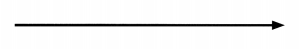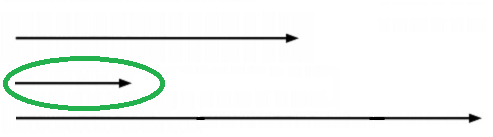Practice the problems of Math in Focus Grade 1 Workbook Answer Key Mid Year Review to score better marks in the exam.

Test Prep
Multiple Choice

Fill in the circle next to the correct answer.

Question 1.
How many stars are there?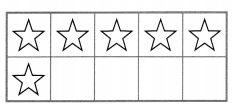(A) 10
(B) 8
(C) 7
(D) 6

Question 2.
Which number is greater than 8?
(A) 8
(B) 10
(C) 7
(D) 0

Question 3.
Which star makes 10?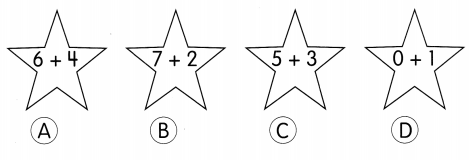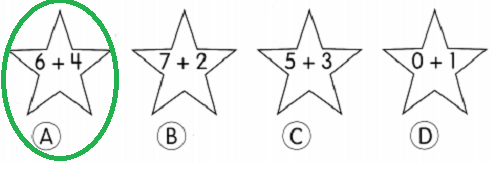Question 4.
Which star makes 1 less than 7?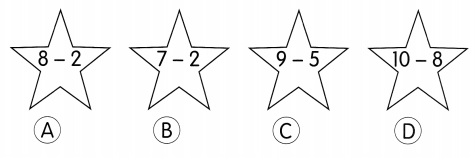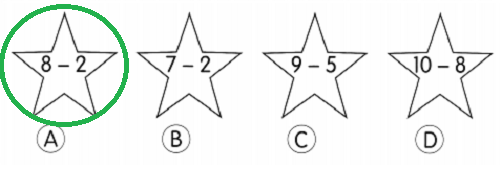Question 5.
Find the missing number.
___ + 9 = 10
(A) 11
(B) 8
(C) 1
(D) 0

Question 6.
Find the missing number.
8 – ___ = 4
(A) 8
(B) 5
(C) 4
(D) 2

Question 7.
How many sides does a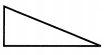have?
(A) 4
(B) 3
(C) 2
(D) 0

Question 8.
How many corners does a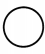have?
(A) 0
(B) 1
(C) 2
(D) 5

Question 9.
How are these shapes sorted?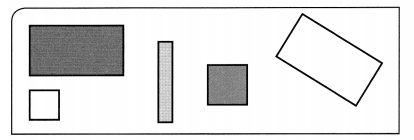(A) shape
(B) color
(C) size
(D) number of sides

Question 10.
Which solid shape can you roll and slide?
(A) sphere
(B) cone
(c) cube
(D) pyramid

Question 11.
Complete the pattern.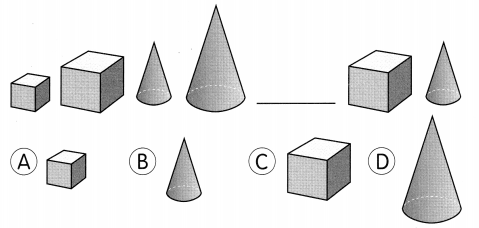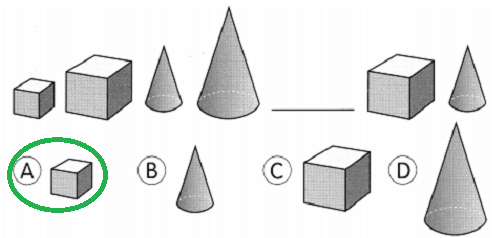Question 12.
In which position is the black bird?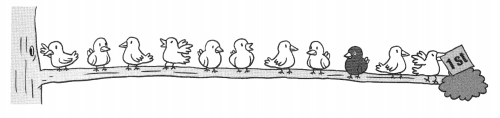Answer: Third position is the black bird.

Question 13.
What do ten and nine make?
(A) 1
(B) 9
(C) 19
(D) 20

Question 14.
16 – 8 = ?
(A) 8
(B) 9
(C) 16
(D) 18

Question 15.
The tennis racket is about ________ shoes long.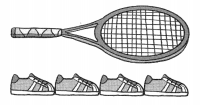(A) 5
(B) 4
(C) 3
(D) 1

Write the numbers in words.

Question 16.
8 _______________

Question 17.
19 _______________

Question 18.
12 _______________

Complete the number patterns.

Question 19.
______ 1, 2, 3, ___, 5
Answer: 0, 1, 2, 3, 4, 5

Question 20.
____, 16, 14, ___, 10, 8 _______
Answer: 18, 16, 14, 12, 10, 8, 6

Question 21.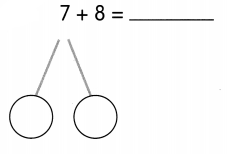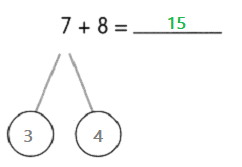Question 22.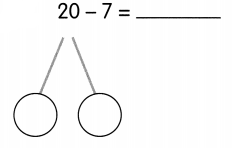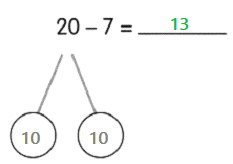Order the numbers from greatest to least.

Question 23.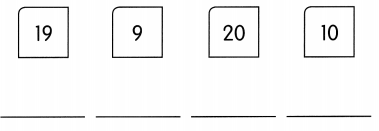Look at the picture. Circle the words for the shapes you see.

Question 24.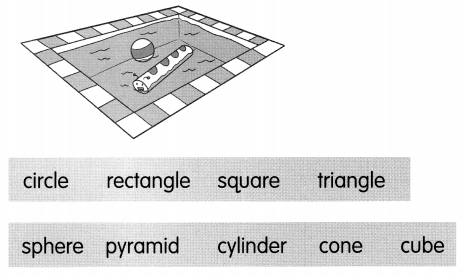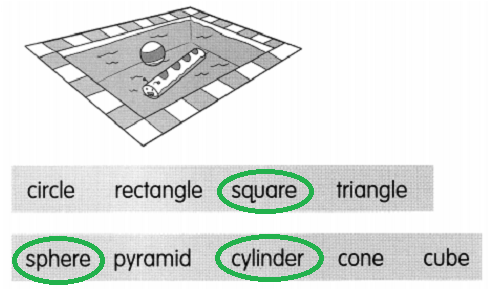Look at the picture.
Then fill in the blanks.
Use the words ¡n the box.

Question 25.
Maria is last from the ____.

Question 26.
Jamal is ______________ on the right.

Question 27.
Liping is ______________ Josh and Jamal.

Question 28.
The mouse is ____ Josh.

Look at the picture. Then fill in the blanks.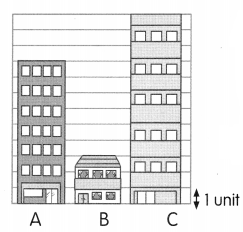Question 29.
Building A is ______ units tall.
Answer: Building A is 9 units tall.

Question 30.
Building B is ______ units tall.
Answer: Building B is 3 units tall.

Question 31.
Building C is _______ units tall.
Answer: Building C is 12 units tall

Question 32.
Building ____ is the tallest.
Answer: Building C is the tallest.

Extended Response

Solve.

Question 33.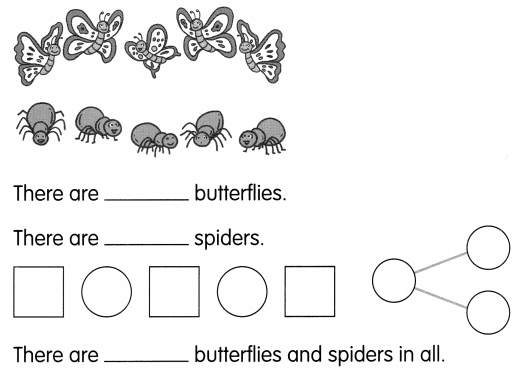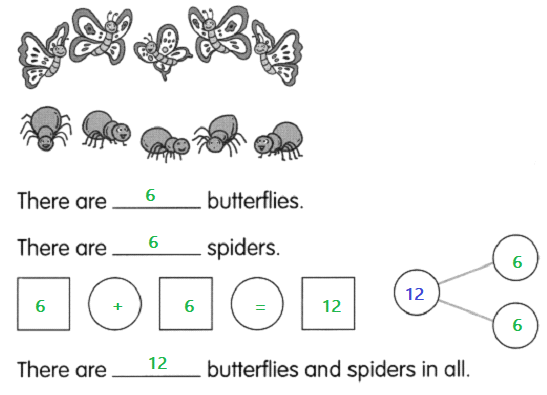Question 34.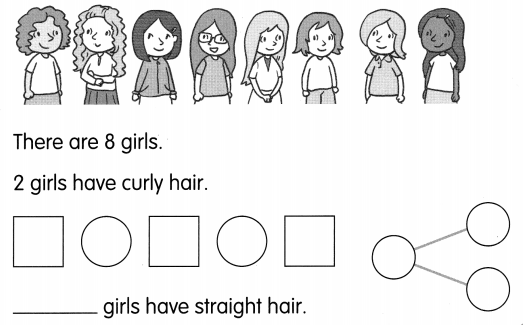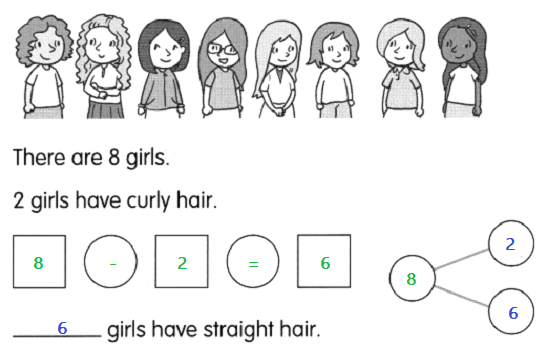Show the number.
Draw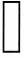for tens and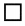for ones. Then fill in the blanks.

Question 35.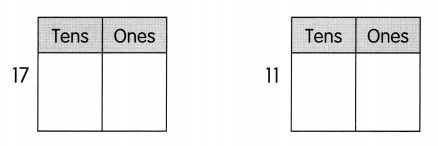_______ is the greater number.
It is greater by ___.
17 is the greater number.
It is greater by 11.

Fill in the blanks.

Question 36.
7 + 8 = ?
7 + 8 is double ___ plus ____
7 + 8 = 7 + ___ plus ____
= _____ + 1
= _____
7 + 8 is double 7 plus 1
7 + 8 = 7 + 7 plus 1
= 14+ 1
= 15

Question 37.
Mom has 13 buttons. She uses some to sew a dress. 7 buttons are left. How many buttons does Mom use?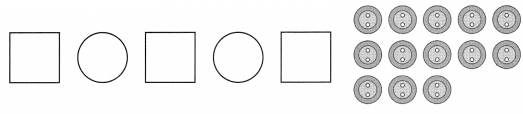Mom uses _______ buttons.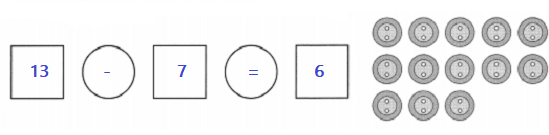Question 38.
Andrew gives away 9 muffins. He has 8 muffins left. How many muffins did he have at first?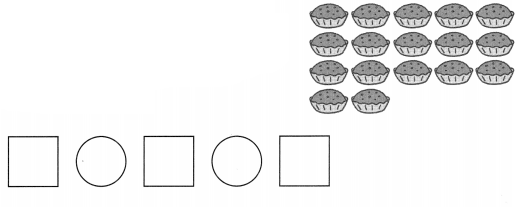Andrew had ________ muffins at first.